## Chapter 9: Search Trees II

### 9.3 Multi-Way Search Trees

Definition
• A node v of an ordered tree is a d-node if v has d children.
• A multi-way search tree is an ordered tree such that:
• Each internal node v has at least two children (d-node with d >= 2 )
• v stores d − 1 key-element items (ki, ei)  (d is the number of children)
• For a node with children v1, v2, …, vd storing keys k1 < k2 < … < kd−1
• keys in the subtree of v1 are less than k1
• keys in the subtree of vi are between ki−1 and ki (i = 2, …, d − 1)
• keys in the subtree of vd are greater than kd−1
• The leaves store no items and serve as placeholders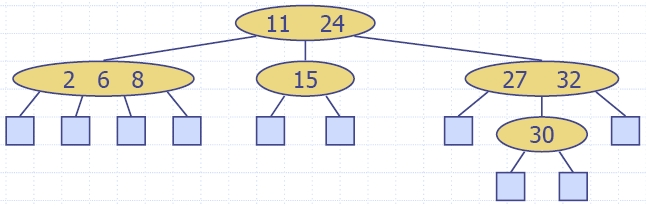• We can extend the notion of inorder traversal from binary trees to multi-way search trees
• Namely, we visit item (ki, ei) of node v between the recursive traversals of the subtrees of v rooted at children vi and vi+1
• An inorder traversal of a multi-way search tree visits the keys in increasing order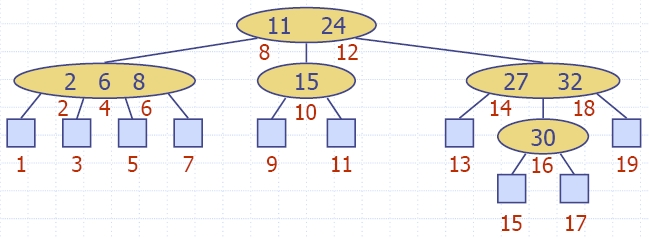Searching in a Multi-Way Tree
• Similar to search in a binary search tree
• For each internal node with children v1, v2, …, vd and keys k1, k2, , kd−1
• k = ki (i = 1, …, d − 1): the search terminates successfully
• k < k1: we continue the search in child v1
• ki−1 < k < ki (i = 2, …, d − 1): we continue the search in child vi
• k > kd−1: we continue the search in child vd
• Reaching an external node terminates the search unsuccessfully
• Example: search for 30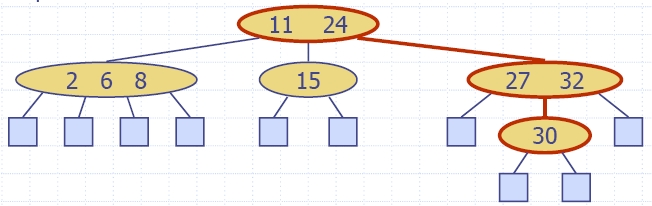### 9.4 (2,4) Trees

• A (2,4) tree (also called 2-4 tree or 2-3-4 tree) is a multi-way search tree with the following properties
• Node-Size Property: every internal node has at most four children (and at least two)
• Depth Property: all the external nodes have the same depth
• Depending on the number of children, an internal node of a (2,4) tree is called a 2-node, 3-node or 4-node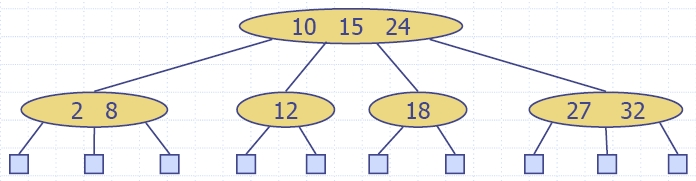• Theorem: A (2,4) tree storing n items has height O(log n)
• Proof:
• Let h be the height of a (2,4) tree with n items
• Since there are at least 2i items at depth i = 0, … , h −1 and no items at depth h, we have
n ≥ 1 + 2 + 4 +… + 2h−1 = 2h − 1
• Thus, h ≤ log (n + 1)
• Searching in a (2,4) tree with n items takes O(log n) time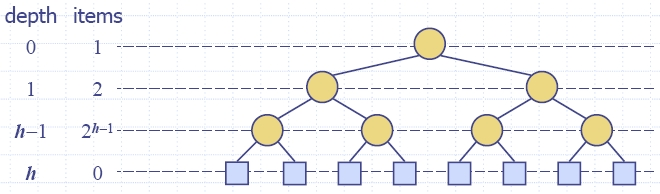9.4.1 Update Operations

Insertion
• We insert a new item (k, e) at the parent v of the leaf reached by searching for k
• We preserve the depth property but
• We may cause an overflow (i.e., node v may become a 5-node)
• Example: inserting key 30 causes an overflow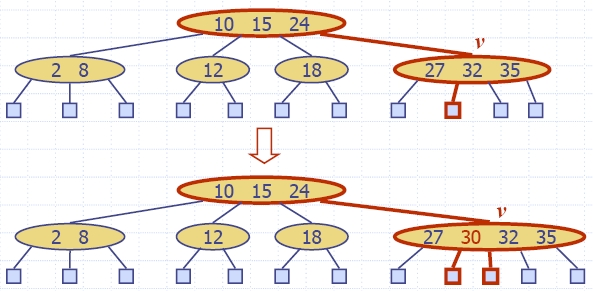• We handle an overflow at a 5-node v with a split operation:
• let v1, , v5 be the children of v and k1, , k4 be the keys of v
• node v is replaced nodes v' and v"
• v' is a 3-node with keys k1, k2 and children v1, v2, v3
• v" is a 2-node with key k4 and children v4, v5
• key k3 is inserted into the parent u of v (a new root may be created)
• The overflow may propagate to the parent node uAlgorithm insertItem(k, e) 1.We search for key k to locate the insertion node v 2.We add the new item (k, e) at node v 3. while overflow(v)         if isRoot(v)             create a new empty root above v         v ← split(v)

Analysis of Insertion in a (2,4) Tree

• Let T be a (2,4) tree with n items
• Tree T has O(log n) height
• Searching takes O(log n) time because we visit O(log n) nodes
• Restoring Node-Size Property takes O(log n) time because each split takes O(1) time and we perform O(log n) splits
• Thus, an insertion in a (2,4) tree takes O(log n) time
Removal
• We reduce deletion of an item to the case where the item is at the node with leaf children
• Otherwise, we replace the item with its inorder successor (or, equivalently, with its inorder predecessor) and delete the latter item
• Example: to delete key 24, we replace it with 27 (inorder successor)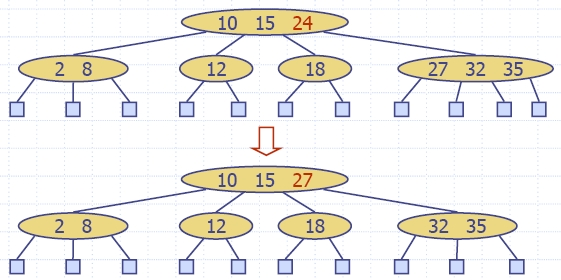• Deleting an item from a node v may cause an underflow, where node v becomes a 1-node with one child and no keys
• To handle an underflow at node v with parent u, we consider two cases:
• Case 1: the adjacent siblings of v are 2-nodes
• Fusion operation:
1. we merge v with an adjacent sibling w
2. we move an item from u to the merged node v'
• After a fusion, the underflow may propagate to the parent u
•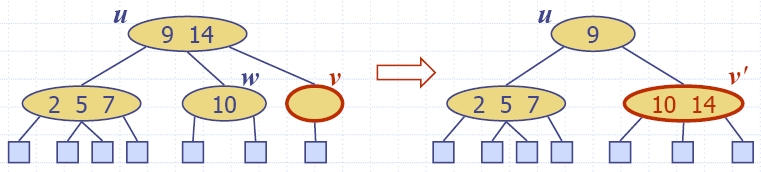• Case 2: an adjacent sibling w of v is a 3-node or a 4-node
• Transfer operation:
1. we move a child of w to v
2. we move an item from u to v
3. we move an item from w to u
• After a transfer, no underflow occurs
•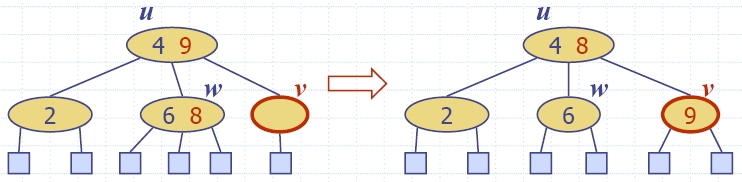Analysis of Removal in a (2,4) Tree
• Let T be a (2,4) tree with n items
• Tree T has O(log n) height
• In a deletion operation
• We visit O(log n) nodes to locate the node from which to delete the item
• We handle an underflow with a series of O(log n) fusions, followed by at most one transfer
• Each fusion and transfer takes O(1) time
• Thus, deleting an item from a (2,4) tree takes O(log n) time

### 9.5 Red-Black Trees

• A red-black tree is a representation of a (2,4) tree by means of a binary tree whose nodes are colored red or black
• In comparison with its associated (2,4) tree, a red-black tree has:
• same logarithmic time performance
• simpler implementation with a single node type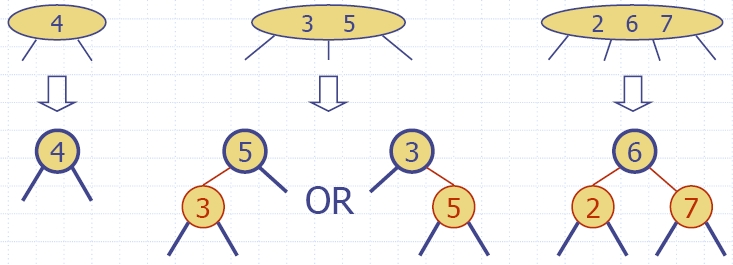• A red-black tree can also be defined as a binary search tree that satisfies the following properties:
• Root Property: the root is black
• External Property: every leaf is black
• Internal Property: the children of a red node are black
• Depth Property: all the leaves have the same black depth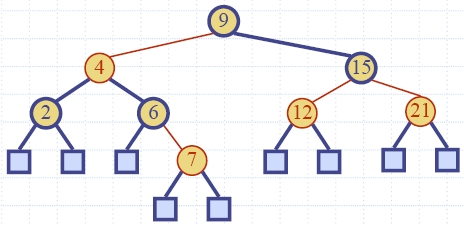• Theorem: A red-black tree storing n items has height O(log n)
• Proof:
• The height of a red-black tree is at most twice the height of its associated (2,4) tree, which is O(log n)
• The search algorithm for a red-black tree is the same as that for a binary search tree
• By the above theorem, searching in a red-black tree takes O(log n) time
9.5.1 Update Operations

Insertion
• To perform operation insertItem(k, e), we execute the insertion algorithm for binary search trees and color red the newly inserted node z unless it is the root
• We preserve the root, external, and depth properties
• If the parent v of z is black, we also preserve the internal property and we are done
• If the parent v of z is red, we have a double red (i.e., a violation of the internal property), which requires a reorganization of the tree
• Example where the insertion of 4 causes a double red: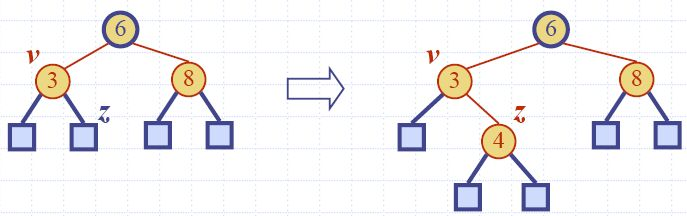• Consider a double red with child z and parent v, and let w be the sibling of v: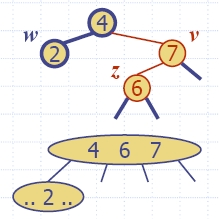Case 1: w is black The double red is an incorrect replacement of a 4-node Restructuring: we change the 4-node replacement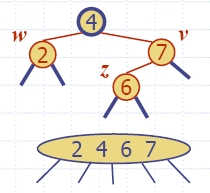Case 2: w is red The double red corresponds to an overflow Recoloring: we perform the equivalent of a split operation in (2,4)-tree

• A restructuring remedies a child-parent double red when the parent red node has a black sibling
• It is equivalent to restoring the correct replacement of a 4-node
• The internal property is restored and the other properties are preserved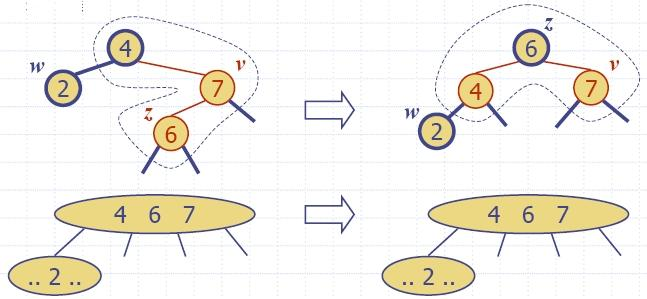• There are four restructuring configurations depending on whether the double red nodes are left or right children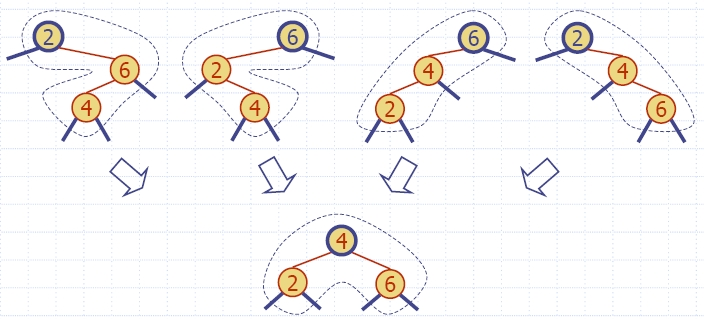• A recoloring remedies a child-parent double red when the parent red node has a red sibling
• The parent v and its sibling w become black and the grandparent u becomes red, unless it is the root
• It is equivalent to performing a split operation on (2,4)-tree on a 5-node
• The double red violation may propagate to the grandparent u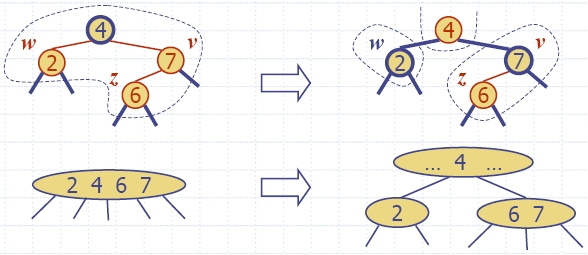Analysis of Insertion in a Red-Black Tree
• Recall that a red-black tree has O(log n) height
• Step 1 takes O(log n) time because we visit O(log n) nodes
• Step 2 takes O(1) time
• Step 3 takes O(log n) time because we perform
• O(log n) recolorings, each taking O(1) time, and
• at most one restructuring taking O(1) time
• Thus, an insertion in a red-black tree takes O(log n) time
Removal
• To perform operation remove(k), we first execute the deletion algorithm for binary search trees
• Let v be the internal node removed, w the external node removed, and r the sibling of w
• If either v or r was red, we color r black and we are done
• If v and r were both black, we color r double black, which is a violation of the depth property requiring a reorganization of the tree
• Example where the deletion of 8 causes a double black: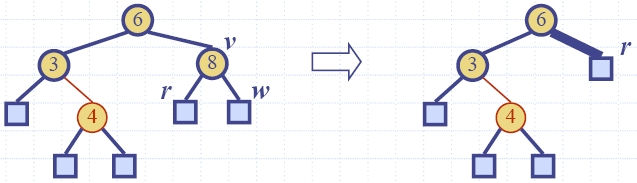• The algorithm for remedying a double black node w with sibling y considers three cases
• Case 1: y is black and has a red child
• We perform a restructuring, equivalent to a transfer, and we are done
• Case 2: y is black and its children are both black
• We perform a recoloring, equivalent to a fusion operation on (2,4)-tree, which may propagate up the double black violation
• Case 3: y is red
• We perform an adjustment, equivalent to choosing a different representation of a 3-node, after which either Case 1 or Case 2 applies
• Deletion in a red-black tree takes O(log n) time

Red-Black Tree Reorganization

Insertion (remedy double red)
 Red-black tree action (2,4) tree action result restructuring change of 4-node representation double red removed recoloring split double red removed or propagated up

Deletion (remedy double black)
 Red-black tree action (2,4) tree action result restructuring transfer double black removed recoloring fusion double black removed or propagated up adjustment change of 3-node representation restructuring or recoloring follows

9.5.2 C++ Implementation
html-9.11 (RBItem)
html-9.12 (RBTree1)
html-9.13 (RBTree2)
html-9.14 (RBTree3)